# Use of Squeezing Theorem to Find Limits

The squeezing theorem, also called the sandwich theorem, is used to find limits.

## Squeezing Theorem.

Ifare functions such that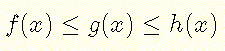for all values of x in some open interval containing a and if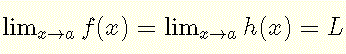then,## Examples with Solutions

### Example 1:

Find the limit:Solution to Example 1:
As
x approaches 0 , 1 / x becomes very large in absolute value and cos(1 / x) becomes highly oscillatory.
However
cos(1 / x) takes values within the interval [-1,1] which is the range of cos x , hence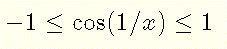Multiply all terms of the above inequality by
x2 (x not equal to 0)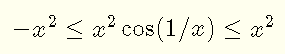The above inequality holds for any value of
x except 0 where $$x^2 \cos (1/x)$$ in undefined. As x approaches 0 both - x2 and x2 approach 0 and according to the squeezing theorem we obtain
$\lim_{x \to 0} x^2 cos(1/x) = 0$

### Example 2:

Find the limit $$\lim_{\to 0} \dfrac{\sin x}{x}$$

Solution to Example 2:
Assume that $$0 \lt x \lt \pi / 2$$ and let us us consider the unit circle, shown below, and a sector
OAC with central angle x in standard position. A is a point on the unit circle and AB is tangent to the circle at point A hence a right angle at this point.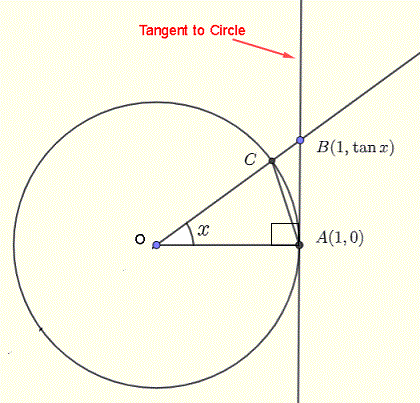Point
C is a point on the unit circle, radius equal to 1, and has coordinates $$(\cos x, \sin x)$$. Let us find the areas of triangle OAC , sector OAC and the right triangle OAB .

Use the
sine formula of the area of a triangle to obtain:
area of triangle $$OAC = (1/2) \sin x \cdot OA \cdot OC = (1/2) \sin x \cdot 1 \cdot 1 = (1/2) \sin x$$
Use the formula of the
area of a sector to obtain:
area of sector $$OAC = (1/2)\cdot(x)\cdot OC^2 = (1/2) x$$
area of right triangle $$OAB = (1/2) \cdot (base) \cdot (height) = (1/2) \cdot (1) \cdot (\tan x) = (1/2) \tan x$$
Comparing, geometrically, the three areas, we can write the inequality $\text{area of triangle} \; OAC \; \lt \; \text{area of sector} \; OAC \; \lt \; \text{area of triangle} \; OAB$ Substitute the areas in the above inequality by their expressions obtained above. $(1/2)(\sin x) \lt (1/2) x \lt (1/2) \tan x$ Multiply all terms by $$\dfrac{2}{\sin x}$$ gives $1 \lt \dfrac{x}{\sin x} \lt 1 / \cos x$ Take the reciprocal and reverse the two inequality symbols in the double inequality $1 \gt \dfrac{\sin x} {x} \gt \cos x$ Which is equivalent to $\cos x \lt \dfrac{\sin x} {x} \lt 1$ It can be shown that the above inequality hols for $$-\pi/ 2 \lt x \lt 0$$ so the the above inequality hold for all $$x$$ except $$x = 0$$ where $$\dfrac{\sin x} {x}$$ is undefined. Since $\lim_{x \to 0} \cos x = 1$ and $\lim_{x \to 0} 1 = 1$ , we can apply the squeezing theorem to obtain $\lim_{x \to 0} \dfrac{\sin x}{x} = 1$ This result is very important and will be used to find other limits of trigonometric functions and derivatives